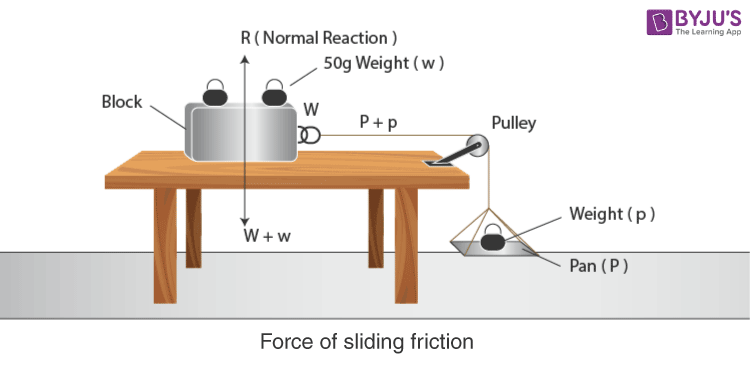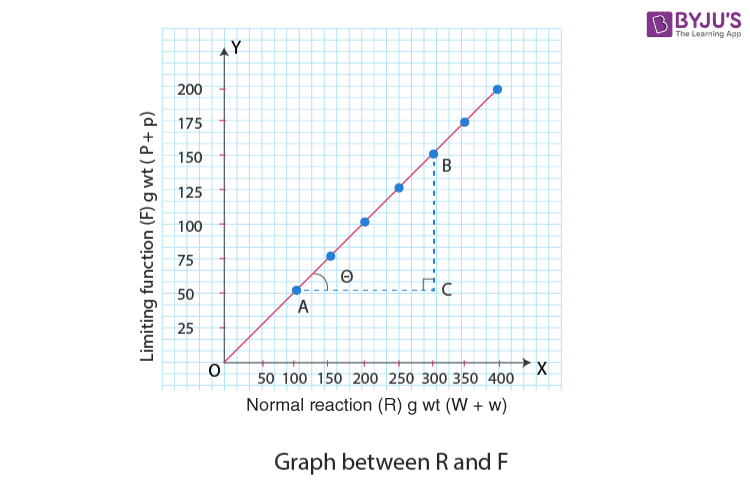# Relationship between the Force of Limiting Friction and Normal Reaction and to Find the Coefficient of Friction between a Block and a Horizontal Surface

We know there exists friction between the two bodies which are in contact with each other, known as static friction. Static friction has a limiting value referred to as limiting friction. It is equal to the least force required to move the body from rest. When the force applied to the two surfaces exceeds the limiting friction, the motion takes place. In this article, let us know in detail the relationship between the force of limiting friction and normal reaction. Also, let us learn to find the coefficient of friction between a block and a horizontal surface.

## Aim

To study the relationship between force of limiting friction and normal reaction and to find the coefficient of friction between a block and a horizontal surface.

## Materials Required

1. Wooden block
2. 50g or 20g weights
3. Horizontal plane fitted with a frictionless pulley at one end
4. Pan
5. Spring balance
7. Spirit level

## Theory

#### What is sliding friction?

Sliding friction is known as the friction between two surfaces when the bodies are sliding over them. It is also known as kinetic friction. Examples of sliding friction are as follows:

• To create heat, both the hands are rubbed together.
• Skis sliding against the snow.
• Sliding of a block across the floor.

#### What is force of sliding friction?

Force of sliding friction is the least force required to make a body slide over the surface.

Force of friction,

$$\begin{array}{l}F\propto R\end{array}$$
$$\begin{array}{l}F=\mu R\end{array}$$

Where,

• μ is the coefficient of friction
• R is the normal reaction
• F is the force of sliding

At equilibrium,

F = P + p

R = W + w

## Diagram## Procedure

1. Check that the pulley is free of friction and also clean the tabletop.
2. Before putting the block on the tabletop, weigh the block.
3. Pass over the block on to the pulley by tieing one end of the thread on the hook of the wooden block.
4. Find the weight of the pan.
5. The pan should be tied to the free end of the thread and left hanging vertically.
6. Put some weights on the pan to pull the block easily.
7. To make the block slide, start tapping the tabletop.
8. Continue tapping by adding the weights little by little.
9. Record the total weights in the pan in the observation table.
10. Repeat steps 8 and 9 by adding one 50g or 20g weight on the wooden block.
11. Every time the weight is increased by 50g or 20, repeat steps 8, 9, and 10 six times.
12. Record the observations as given below.

## Observations

Weight of wooden block, W = ……g wt

Weight of pan, P = ……g wt

 Sl.no Weights on a wooden block (w) in g wt The total weight being pulled (W+w)= Normal reaction in g wt Weight on the pan (p) in g wt Total weight pulling the block and weights (P+p)=limiting friction (F) in g wt 1. 2. 3. 4.

## Calculations

Total weight pulling the block and weights gives the value of the force of sliding friction.

On a horizontal surface, total weights being pulled give normal reaction R. Total weight pulling these weights gives dynamic friction F.

Plot a graph between normal reaction R and limiting friction F, taking R along x-axis and F along the y-axis.

The graph comes to be a straight line as shown below:## Result

It is found that as the total weight pulled increases, the force of limiting friction also increases. The increase is in direct proportion.

The graph shows that limiting friction F is directly proportional to the normal reaction R. it is an agreement with the law of limiting friction.

The constant ratio of FIR is called the coefficient of friction (p). It can be calculated by finding the slope of the graph.

In ΔABC,

Slope of straight line AB = tan Ө =

$$\begin{array}{l}\frac{BC}{AC}=\frac{F}{R}=\frac{150-50}{300-100}\end{array}$$

tan Ө =

$$\begin{array}{l}\frac{F}{R}=\frac{100}{200}=\frac{1}{2}=0.5\end{array}$$
………..(1)

But,

$$\begin{array}{l}F=\mu R\end{array}$$
………………(2)

$$\begin{array}{l}\frac{F}{R}=\mu\end{array}$$

From equation (1) and (2),

$$\begin{array}{l}\mu=tan\theta =0.5\end{array}$$
$$\begin{array}{l}\mu=0.5\end{array}$$

## Precautions

1. The table-top should be horizontal.
2. The thread between block and pulley should be horizontal.
3. The pan should not oscillate or rotate and the weight should be added in small quantities.
4. The tapping of the tabletop should be done gently.
5. The frictionless pulley should be used.

## Sources Of Error

1. The tabletop is not horizontal.
2. The thread between block and pulley may not be horizontal.
3. The frictionless pulley is not used.

## Viva Questions

Q1

### Why is the thread part between block and pulley kept horizontal?

It is kept horizontal for the equal distribution of the total weight of pan and weights.
Q2

### Why is a frictionless pulley being used?

By using a frictionless pulley the overall effective value can be reduced.
Q3

### Why do roads become slippery after rains?

Friction between the feet and road reduces when there is a thin layer of rainwater between them. So the roads become slippery after rains.
Q4

### Why are brake surfaces kept flat?

The surface of the brake are flat because when the brake is pressed, it gets pushed from hydraulic pistons and the surface of the brake rotor. Since it is getting pushed from both sides, friction is created and so the surface should be flat.

Give some examples for sliding friction
Q5

### Give some examples for sliding friction

Examples for sliding friction are:

• Hands are rubbed together, to produce heat.
• Skis sliding against the snow.
• Sliding of a block across the floor.
Hope you have learned about the relationship between the force of limiting friction and normal reaction. Stay tuned with BYJU’S to learn more about other Physics related experiments.
Test Your Knowledge On To Study The Relationship Between Force Of Limiting Friction And Normal Reaction And To Fill The Coefficient Of Friction Between A Block And A Horizontal Surface!